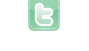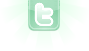## Source code for bouncing ball

1
2
I am very new to greenfoot. I want to make a ball bounce up and down repeatedly when I hit the act button. I have the code to get the ball to bounce down. However, I need the code to get it to bounce up. Can anyone help me out?
Start by posting the code you have! Either upload the scenario (with source code) and post a link to it here, or post the code here directly.
public class Ball extends Actor { /** The speed of the ball. */ private double speed; /** * If we're above the ground, drop the ball. */ public void act() { if(getOneIntersectingObject(Ground.class)==null) { Ground ground = (Ground)getWorld().getObjects(Ground.class).get(0); int max = ground.getY()-getY()-getImage().getHeight()/2-ground.getImage().getHeight()/2; if(speed>max) { speed = max; } setLocation(getX(), (int)(getY()+speed)); speed += 0.1; } else { speed = 0; } } }
I think that if you change the last statement to 'speed = -speed;' (that is negate the value of speed), your ball will bounce infinitely. Negating the value of speed, in essence, says to reverse the direction of motion with the same energy that it found the ground with. Try it out and let me know if it works.
I think that if you change the last statement to 'speed = -speed;' (...), your ball will bounce infinitely.
Not quite; you also need to continue moving. In pseudo code it currently is a bit like: if (not touching the ground) adjust speed move else reverse speed So what happens after you reverse speed? Well, you'll still be touching the ground in the next act() cycle... and so you'll reverse speed again. And so on. Also, I'm not sure that the limitation placed on the speed (int max=....) will necessarily allow the ball to actually intersect the ground - it might stop it, just above the ground.
Ok, I tried what you just said davmac and it won't let me compile it...it says if (not touching the ground) is not a statement
{ if (not touching the ground) adjust speed move else reverse speed } that is how i coded it...what did i do wrong?
No, no, that was pseudo-code - not real Java code. Sorry for the confusion. "pseudo code" means not real code, but something that is halfway between code and plain English. It won't compile, but it is usually easier to understand (for a human). In any case the pseudo code was wrong, which was my main point. Where you have:
```        else {
speed = 0;
}```
You need to do as danpost suggested - but then you also need to move the ball (according to the speed). You already have the code to do that above - you can copy and paste one line...
ok I did what danpost suggested. What do I copy and paste to make the ball bouce back up.
The line that sets the actor location! That is, the line that actually causes the ball to move. You have it already, you just need to copy it into the "else" block.
public void act() { if(getOneIntersectingObject(Ground.class)==null) { Ground ground = (Ground)getWorld().getObjects(Ground.class).get(0); int max = ground.getY()-getY()-getImage().getHeight()/2-ground.getImage().getHeight()/2; if(speed>max) { speed = max; } setLocation(getX(), (int)(getY()+speed)); speed += 0.1; } else { setLocation(getX(), (int)(getY()+speed)); speed = -speed; } ****The ball will not bounce back up...I told you I really do not know what I'm doing with this. This is extremely frustrating.
Reverse the two statements in the else,and add another speed += 0.1 statement, like so:
```else {
speed = -speed;
setLocation(getX(), (int)(getY() + speed));
speed += 0.1;
}```
The condition for the if was that if not intersecting the ground, move some and add speed due to gravity, else (if intersecting the ground ) reverse direction and move in THAT direction, still adding gravity (adding to a negative value is like slowing it down while it rises up). As far as your ball not bouncing, 1) Initialize the location of the ball at its highest point. 2) Initialize the speed value to zero (0). If both these are complied with, let us know. Also, if you still cannot get the ball to bounce, I will run a test scenario.
public void act() { if(getOneIntersectingObject(Ground.class)==null) { Ground ground = (Ground)getWorld().getObjects(Ground.class).get(0); int max = ground.getY()-getY()-getImage().getHeight()/2-ground.getImage().getHeight()/2; if(speed>max) { speed = max; } setLocation(getX(), (int)(getY()+speed)); speed += 0.1; } else { speed = -speed; setLocation(getX(), (int)(getY()+speed)); speed += 0.1; } That's what I changed it to. I'm sorry but I'm confused about what you're saying if the ball is not bouncing. I am a beginner at this and trying to understand it more my class.
I wrote a simple scenario that just has a bouncing ball. I uses the edge of the world to bounce on (not an object). By the way, what does it mean 'if(speed>max) { speed = max; }'? SPEED = the velocity of the ball and MAX is the location at which the ball bounces, supposedly. I say supposedly because in your 'int max =' statement you have '-getY()', which is the current vertical location of the ball, which should have nothing to do with where the bounce occurs. What I have for a edge of world (height of 400) bounce is this:
```public class Ball extends Actor
{
// Ball placed at bounce-point with speed consistant with that after bounce

private double speed = -9.0;

public void act()
{
int myMaxY = getWorld().getHeight() - getImage().getHeight() / 2;
int myNewY = getY() + (int) speed;
// Check to see if edge of world will be reached on next move
// I used the world's edge here, but it could be changed to bounce on an object instead (like the Ground)
if ( myNewY > myMaxY)
{
// Adjust the value for the new location of ball after the bounce
myNewY = 2 * myMaxY - myNewY;
// Set ball's location at bounce-point
setLocation(getX(), myMaxY);
// Display ball bouncing
getWorld().repaint();
// Set ball's location at new point after bounce
setLocation(getX(), myNewY);
// Apply bounce (-) and gravity (0.1) to speed
speed = 0.1 - speed;
}
else
{
// Move ball to new location
setLocation(getX(), myNewY);
// Apply gravity (0.1) to speed
speed += 0.1;
}
}
}```
For a bounce off an object, change 'getWorld().getHeight()' to 'ground.getY() - ground.getImage().getHeight() / 2' after getting 'ground' as you have in your code.
Where would i put that in my code? By the way...thanks guys for all of your help!
There are more replies on the next page.
1
2